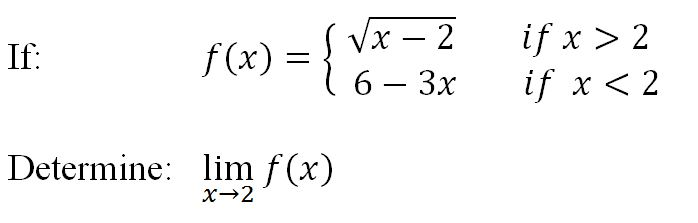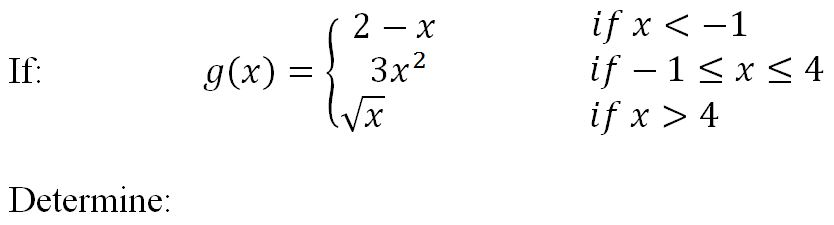# Finding limits algebraically - direct substitution

### Finding limits algebraically - direct substitution

Graphically finding the limit of a function is not always easy, as an alternative, we now shift our focus to finding the limit of a function algebraically. In this section, we will learn how to apply direct substitution to evaluate the limit of a function.

#### Lessons

• if: a function $f$ is continuous at a number $a$
then: direct substitution can be applied: $\lim_{x \to a^-} f(x) = \lim_{x \to a^+} f(x) =\lim_{x \to a} f(x)= f(a)$

• Polynomial functions are continuous everywhere, therefore "direct substitution" can ALWAYS be applied to evaluate limits at any number.
• Introduction
No more finding limits "graphically"; Now, finding limits "algebraically"!
a)
What is Direct Substitution?

• 1.
Evaluate the limit:
a)
$\lim_{x \to 3} (5x^2-20x+17)$

b)
$\lim_{x \to -2} \frac{{{x^3} + 3{x^2} - 1}}{{5 - 4x}}$

c)
$\lim_{x \to 0}\left| x \right|$

d)
$\lim_{x \to \frac{\pi }{2}} \;\frac{{\sin x}}{{2 - \cos x}}$

• 2.
Evaluate the one-sided limit:
a)
$\lim_{x \to 3^-} (5x^2-20x+17)$
$\lim_{x \to 3^+} (5x^2-20x+17)$

b)
$\lim_{x \to {4^ - }} \sqrt {x - 4}$
$\lim_{x \to {4^ + }} \sqrt {x - 4}$

• 3.• 4.a)
$\lim_{x \to {-1^ - }} g(x)$
$\lim_{x \to {-1^ + }} g(x)$
$\lim_{x \to {-1}} g(x)$

b)
$\lim_{x \to {4^ - }} g(x)$
$\lim_{x \to {4^ + }} g(x)$
$\lim_{x \to {4}} g(x)$